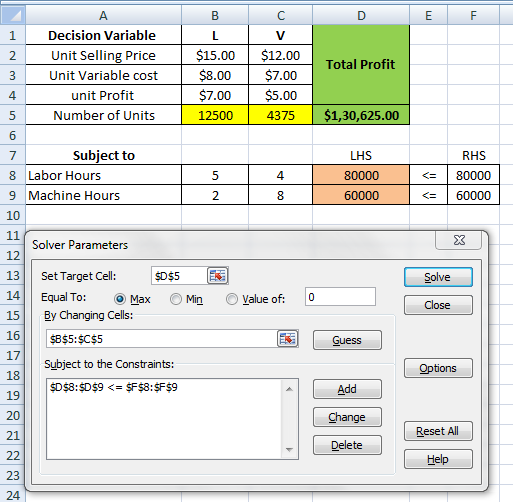# Question & Answer: Betty DeRose, Inc. produces and sells two products, L and V. Revenue and cost…..

Betty DeRose, Inc. produces and sells two products, L and V. Revenue and cost information for the two products from last month appear below:

Product L      Product V

Don't use plagiarized sources. Get Your Custom Essay on
Question & Answer: Betty DeRose, Inc. produces and sells two products, L and V. Revenue and cost…..
GET AN ESSAY WRITTEN FOR YOU FROM AS LOW AS \$13/PAGE

selling price per unit ………..    \$15.00         \$12.00

variable costs per unit ……….    \$ 8.00         \$ 7.00

For the coming month, Betty would like to use linear programming in order
to maximize monthly profits.

Each month Betty has 80,000 direct labor hours available and 60,000 machine
hours available. Product L requires 5 direct labor hours for each unit and
2 machine hours for each unit. Product V requires 4 direct labor hours for
each unit and 8 machine hours for each unit.

Calculate the number of units of Product L that should be produced in order
to maximize net income
Do not use the \$, decimals, or type the word units
.

Decision Variables:

L = units of product L to be produced

V = units of product V to be produced

Objective Function:

Objective is to maximize the monthly profit. The profit is given as follows:

Profit = unit Selling price – unit Variable cost

The objective or profit function is given as follows:

Max. Z = (15 – 8)L + (12 – 7)V

Max. Z = \$7L + \$5V

Subject To:

1) Available direct labor hours are 80,000 hours per month. Product L and V requires 5 and 4 hours respectively

5L + 4V <= 80,000

2) Available machine hours are 60,000 hours per month. Product L and V requires 2 and 8 hours respectively

2L + 8V <= 60,000

3) Non-negativity constraint:

L, V >= 0

Excel Formulation and Solver Solution:

Copy paste following table in excel:

 Decision Variable L V Total Profit Unit Selling Price 15 12 Unit Variable cost 8 7 unit Profit =+B2-B3 =+C2-C3 Number of Units 12500 4375 =SUMPRODUCT(B3:C3,\$B\$5:\$C\$5) Subject to LHS RHS Labor Hours 5 4 =SUMPRODUCT(\$B\$5:\$C\$5,B8:C8) <= 80000 Machine Hours 2 8 =SUMPRODUCT(\$B\$5:\$C\$5,B9:C9) <= 60000Optimal Solution:

Unit of Product L = 12,500 units

Units of Product L = 4,375

Total Profit = \$130,625